# Active sampling

Experimentalists often ask “how many responses should I collect with Mechanical Turk?” It’d be nice to obtain high quality embeddings with few human responses, both to reduce expenses and to ask informative questions for humans.

Active sampling decides which questions to ask about, instead of asking random questions. This can mean that higher accuracies are reached sooner, or that less human responses are required to reach a particular accuracy. This might enable you to ask about more items.

Active sampling algorithms are difficult in this “triplet embedding” context, especially when in the crowdsourcing setting. However, Salmon enables good performance of active sampling algorithms in crowdsourcing settings. Specifically, there is evidence that Salmon provides the following features:

1. Salmon’s active sampling works well for any (practical) number of targets $$n$$.

2. Random sampling requires about 2–3× more human responses than Salmon’s active sampling.

3. Even if responses are received very quickly, Salmon’s active sampling (almost always) performs no worse than random sampling.

Simulations have been run for each of these points. To show these points, let’s first walk through the experimental setup before detailing how Salmon’s active sampling performs when compared with previous work. 2 Then, let’s investigate how changing the number of targets $$n$$ and the response rate affect the embeddings.

Warning

All results on this page are for a specific dataset with simulated human responses. Other papers provide evidence of active gains; 1 however, they are more moderate than the results here.

Note

Generally, the number of responses required to reach a certain embedding quality scales like $$nd\log_2(n)$$. 3 4 For more detail, see the FAQ “How many responses will be needed?.”

## Setup

To illustrate the difference between random and active sampling, let’s run some experiments with the “alien eggs” dataset. For $$n=30$$ objects, that dataset looks like the following: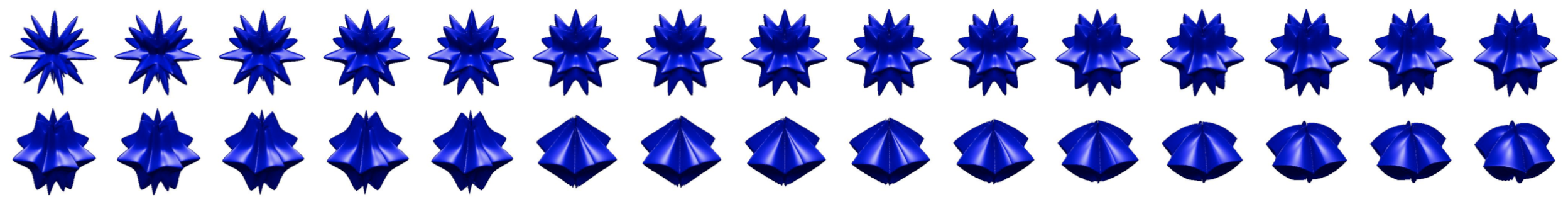This dataset is characterized by one parameter, the “smoothness” of each egg, so they have a 1D embedding. However, let’s embed into $$d=2$$ dimensions to simulate a mistake and to mirror prior work. 2 This page will be concerned with the data scientist workflow, and every experiment below will use the same workflow a data scientists would:

1. Launch Salmon.

2. Simulate human users. 8

4. Generate the embedding offline.

Every graph shows points with this data flow. Each point shown only changes the number of responses available or the sampling method used. 5 Unless explicitly mentioned, let’s compare random and active sampling with these init.yaml configurations:

d: 2
samplers:
ARR: {random_state: 42}  # active or adaptive sampling
Random: {}  # random sampling


The “ARR” stands for “asynchronous round robin” and creates an instance of ARR. For this class, the query head is randomly chosen, and then for each head, the best comparison items are ranked by some measure (information gain by default).

Note

This page shows results of experiments run with Salmon. For complete details, see https://github.com/stsievert/salmon-experiments

## Baseline

First, let’s run a basic experiment, one that will very closely mirror prior work: 2 let’s take the $$n= 30$$ objects above and embed them into $$d=2$$ dimensions. To mirror their setup, let’s develop a noise model from their collected responses and submit responses at the same time as their responses. Let’s do this many times, and generate a graph of how many responses are required to reach a particular accuracy: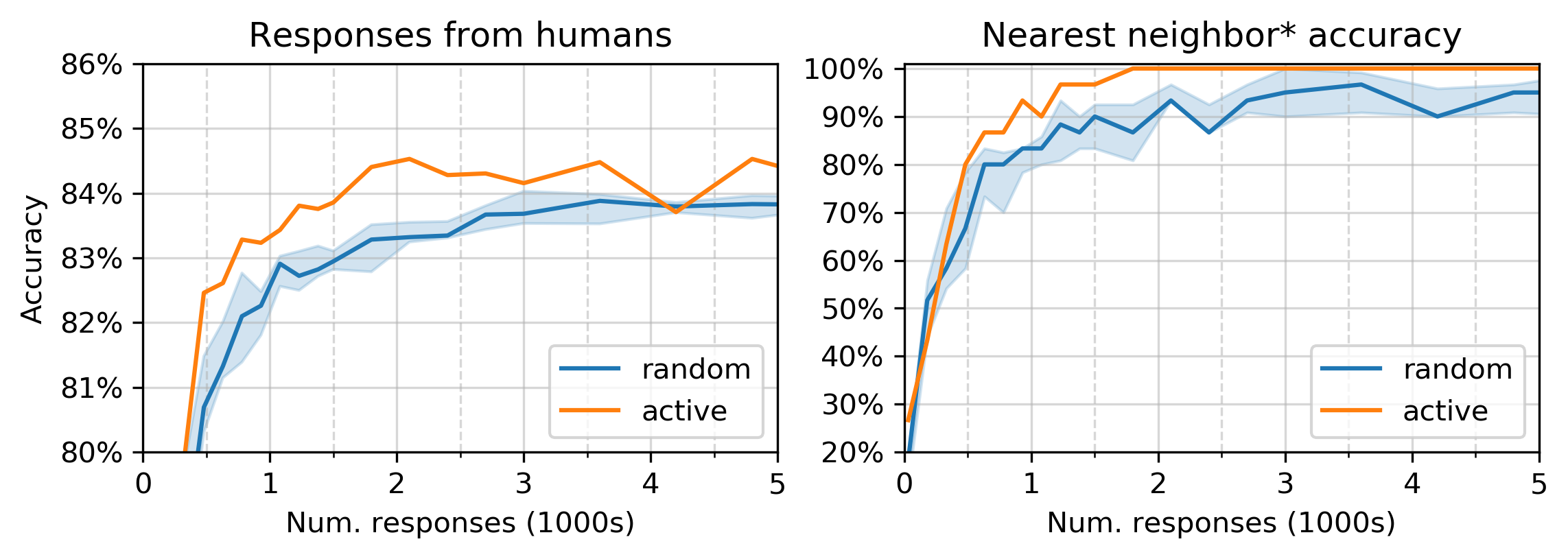This graph uses the same test set as the NEXT paper, which (mis)defines “nearest neighbor accuracy” as “is the true nearest neighbor one of the three closest objects?” 2 (the reason for the * in the title). 7

### Embedding quality

Experimentalist often cares about the underlying structure more than the accuracy. To start, let’s assume that there’s no clear relationship between items. Then, this visualization is most appropriate for the embeddings of particular accuracies: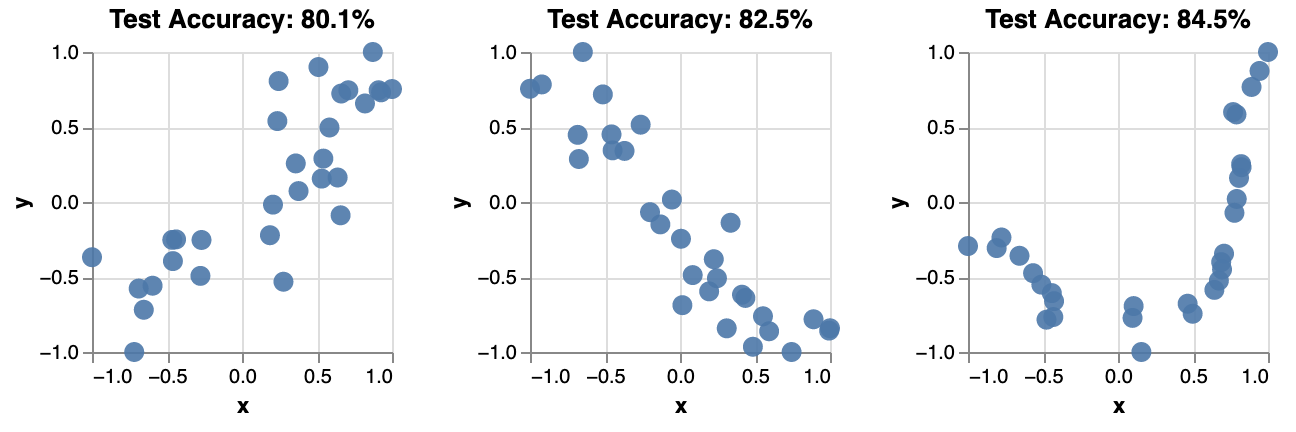These embeddings are remarkably simple, and have a clear and known relationship. Because of that, let’s show the embeddings with colors from now on: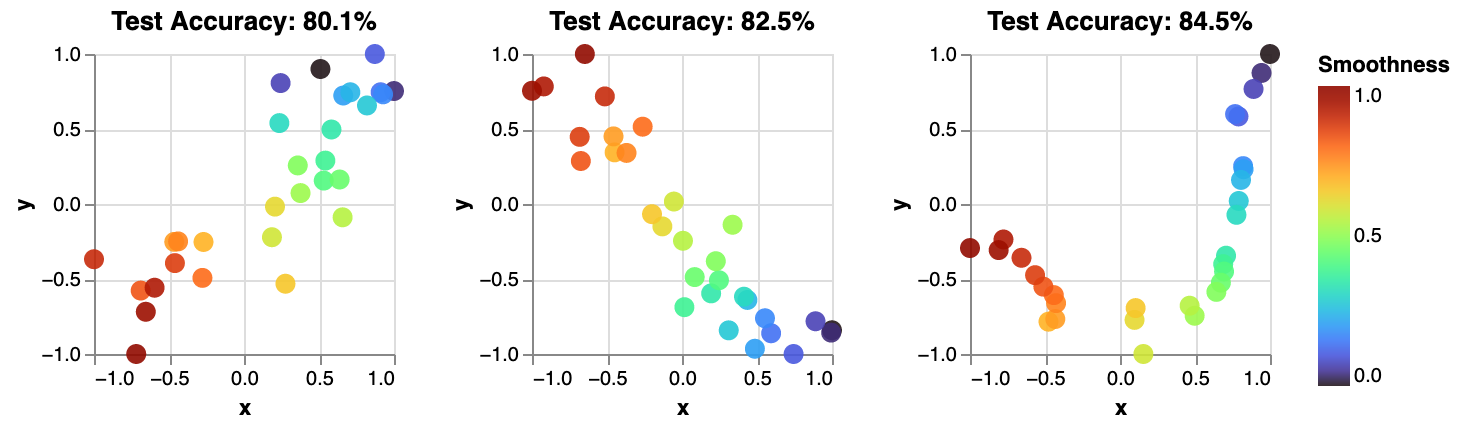Note

Only relative distances matter in these embeddings. It doesn’t matter how the embedding is rotated, or how the axes are scaled.

## Number of targets

Users of Salmon frequently have a variable number of target items. For example, they might be asking about colors – a continuous space, so they can easily change the “number of targets.” So, how does the number of targets influence embedding quality?

To examine that, let’s run the same experiment above, but with 30, 90, 180 and 300 “alien eggs.” Here’s the number of responses required to reach a particular accuracy to simulated human responses: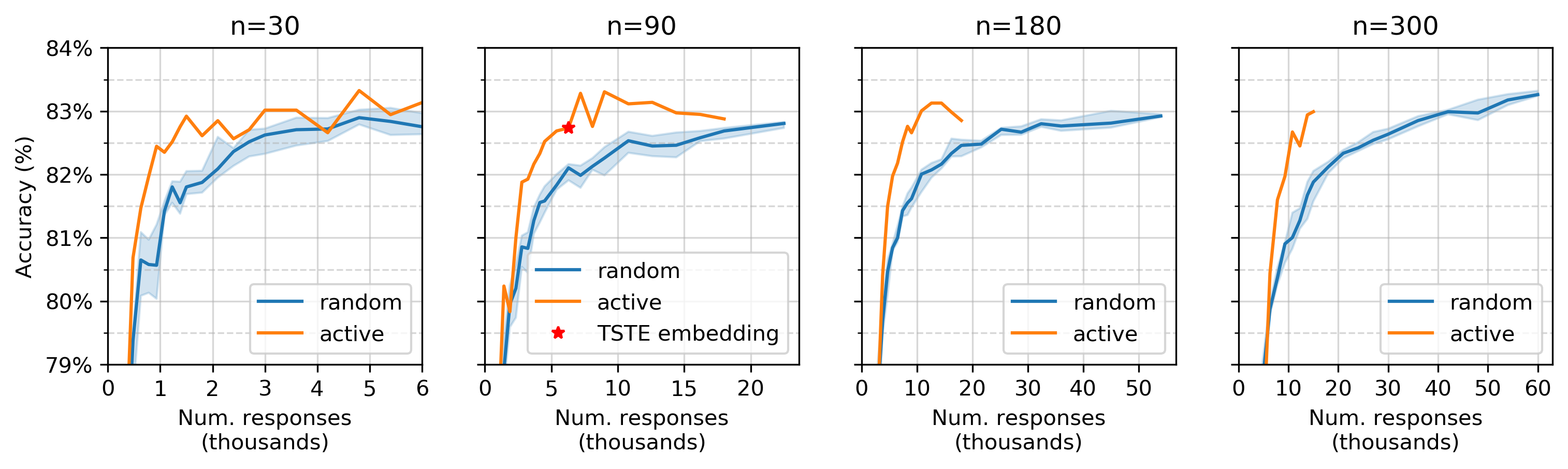The accuracy of simulated human responses for various number of responses. The shaded region represents the 25–75% percentile among 10 runs, and the solid line represents the median. The y-axis labels are shared with all plots.

### Embedding quality

Here’s the underlying embeddings for $$n = 180$$ for various accuracy levels on simulated human responses: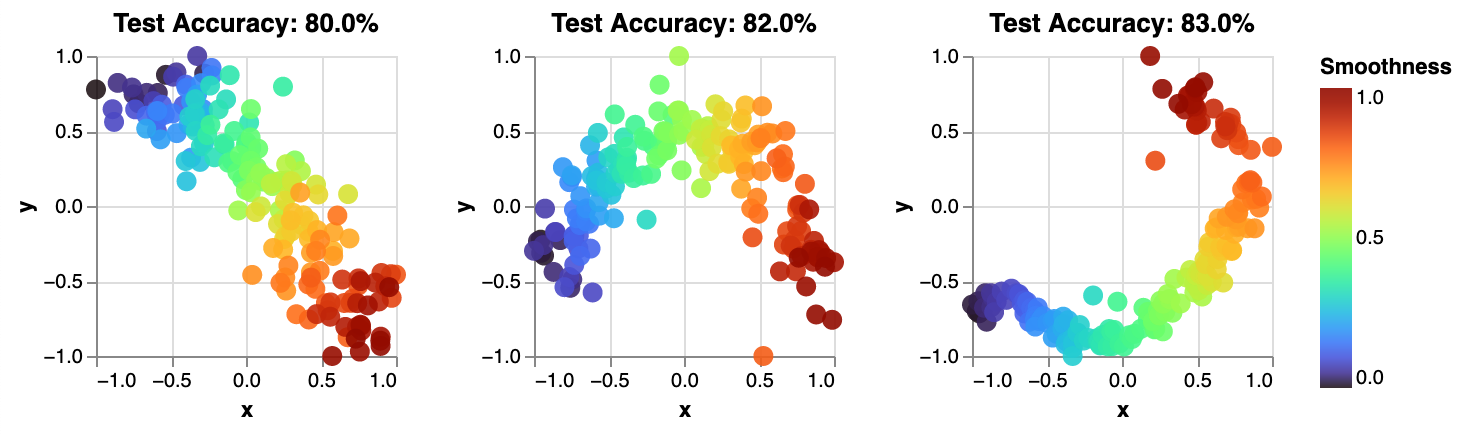“Test accuracy: 80%” means “80% accurate on simulated human responses not used for training.” The local accuracy gets much better as accuracy increases. To visualize the structure of the underlying embedding, let’s look at the average items closer than the true nearest neighbor. The smaller this value is, the smoother the color gradient is above.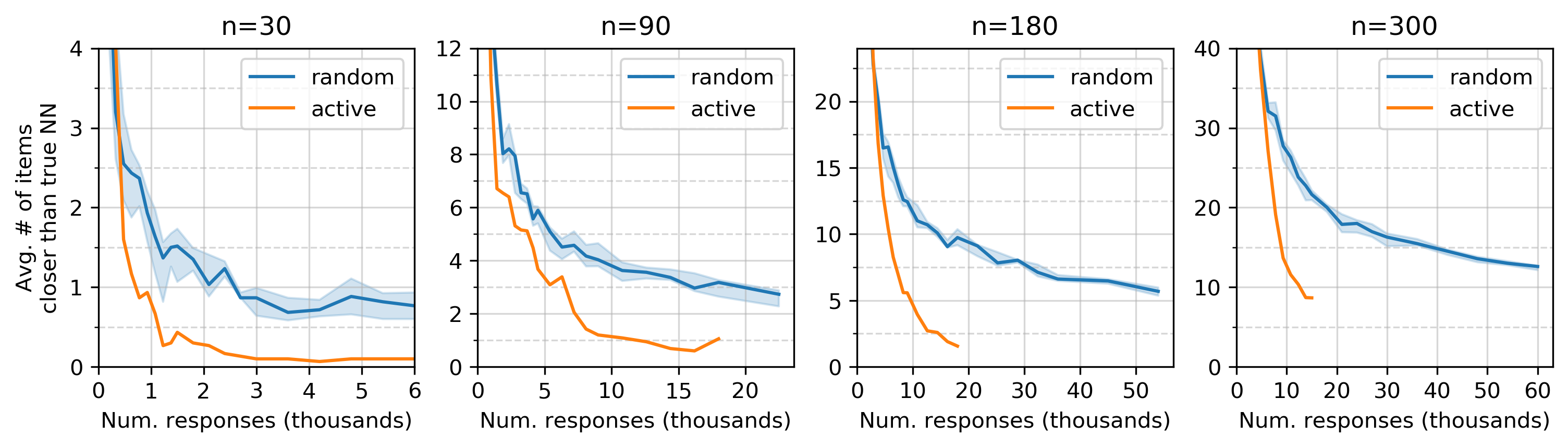The average number of items closer than the true nearest neighbor. The upper limit on the y-axis represents a very moderately accurate embedding, slightly worse than the 80% accurate embedding above. The shaded region/solid line has the same meaning as above, the interquartile range and median.

If the embedding were a 1D manifold but not quite perfect, 9 the value on this plot would be 0.5. As with accuracy, there’s a clear advantage to active sampling – active sampling requires a lot fewer responses to obtain a high quality embedding in this simulation.

## Response rate

One detail has been swept under the rug: the rate at which Salmon received responses. There would be no gain from adaptive algorithms if all 10,000 responses were received in 1 second. In fact, the response rate above is variable: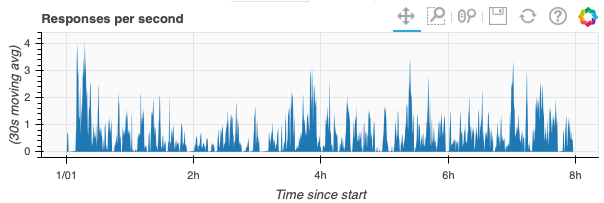Here’s a summary of the server side timings: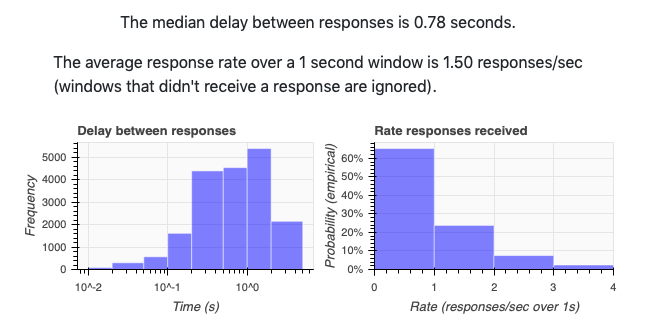How does this variable response rate affect adaptive gains? Let’s run the same data flow as above, but with a constant response rate and (functionally) Salmon v0.6.0. In this experiment, the number of users varies between 1 concurrent user to 10 concurrent users with a mean response time of 1 second. Here’s the performance we see for $$n=30$$ alien eggs (the same setup as in Baseline).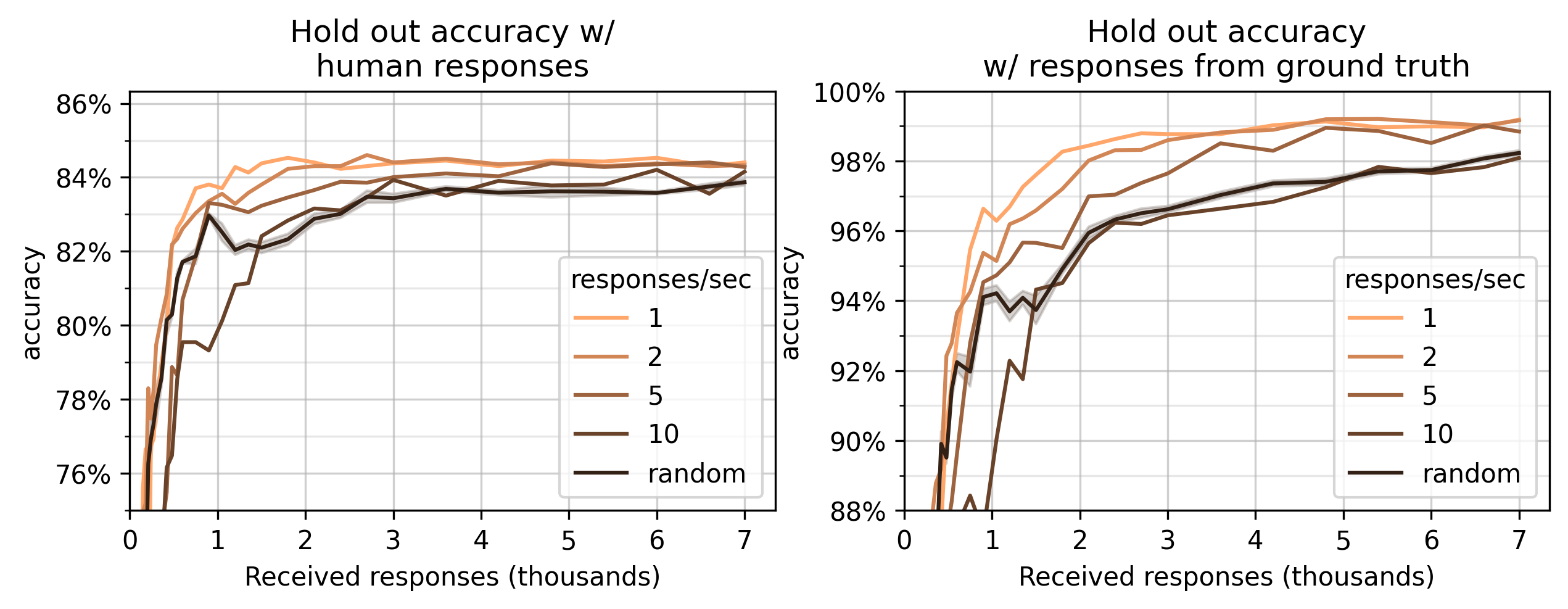This graph shows two measures: accuracy on a set of test human responses (left) and responses that are 100% accurate on the ground truth dataset (right). The graph on the right is a measure of quality on the underlying embedding. The graph on the left shows that that this quality is reflected in hold-out performance on human responses.

These experiments provide evidence that the adaptive sampling above works well in crowdsourcing settings. Additionally, they provide evidence that Salmon’s adaptive sampling does not perform worse than random sampling.

This measure provides evidence that Salmon’s active sampling approach outperforms random sampling. If true, this is an improvement over existing software to deploy triplet queries to crowdsourced audiences: in NEXT’s introduction paper, 2 the authors found “no evidence for gains from adaptive sampling” for (nearly) the same problem. 6

References

1

“Active Perceptual Similarity Modeling with Auxiliary Information” by E. Heim, M. Berger, and L. Seversky, and M. Hauskrecht. 2015. https://arxiv.org/pdf/1511.02254.pdf

2(1,2,3,4,5,6)

“NEXT: A System for Real-World Development, Evaluation, and Application of Active Learning” by K. Jamieson, L. Jain, C. Fernandez, N. Glattard and R. Nowak. 2017. http://papers.nips.cc/paper/5868-next-a-system-for-real-world-development-evaluation-and-application-of-active-learning.pdf

3

“Finite Sample Prediction and Recovery Bounds for Ordinal Embedding.” Jain, Jamieson, & Nowak, (2016). https://papers.nips.cc/paper/2016/file/4e0d67e54ad6626e957d15b08ae128a6-Paper.pdf

4

“Low-dimensional embedding using adaptively selected ordinal data.” Jamieson, Nowak (2011). https://homes.cs.washington.edu/~jamieson/resources/activeMDS.pdf

Footnotes

5

For random sampling, the order is also shuffled (not the case for active).

6

Both experiment use $$n=30$$ objects and embed into $$d=2$$ dimensions. The human noise model used in the Salmon experiments is generated from the responses collected during NEXT’s experiment. The are the same experiment, up to different responses (NEXT actually runs crowdsourcing experiments; Salmon’s noise model is generated from those responses).

7

Astute observers might notice that the accuracy doesn’t perform well when directly compared with NEXT’s results. However, the developed noise model doesn’t exactly mirror the human responses; it’s about 1.5% less accurate (shown below). However, the nearest neighbor accuracy is a measure of the underlying embedding, and Salmon perform better than the NEXT results.

8

Specifically, with a noise model developed the human responses collected for Fig. 3 of the NEXT paper. 2

9

“Not quite perfect” means “1D manifold with a constant distance to the nearest neighbor: an embedding with coordinates [[1, 0], [2, 0], [3, 0], ..., [n - 1, 0]].